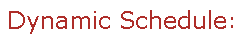Visit Minerva Class Schedule for course dates & times.

# MATH 323 Probability (3 credits)

Offered by: Mathematics and Statistics (Faculty of Science)

### Overview

Mathematics & Statistics (Sci) : Sample space, events, conditional probability, independence of events, Bayes' Theorem. Basic combinatorial probability, random variables, discrete and continuous univariate and multivariate distributions. Independence of random variables. Inequalities, weak law of large numbers, central limit theorem.

Terms: Fall 2022, Winter 2023